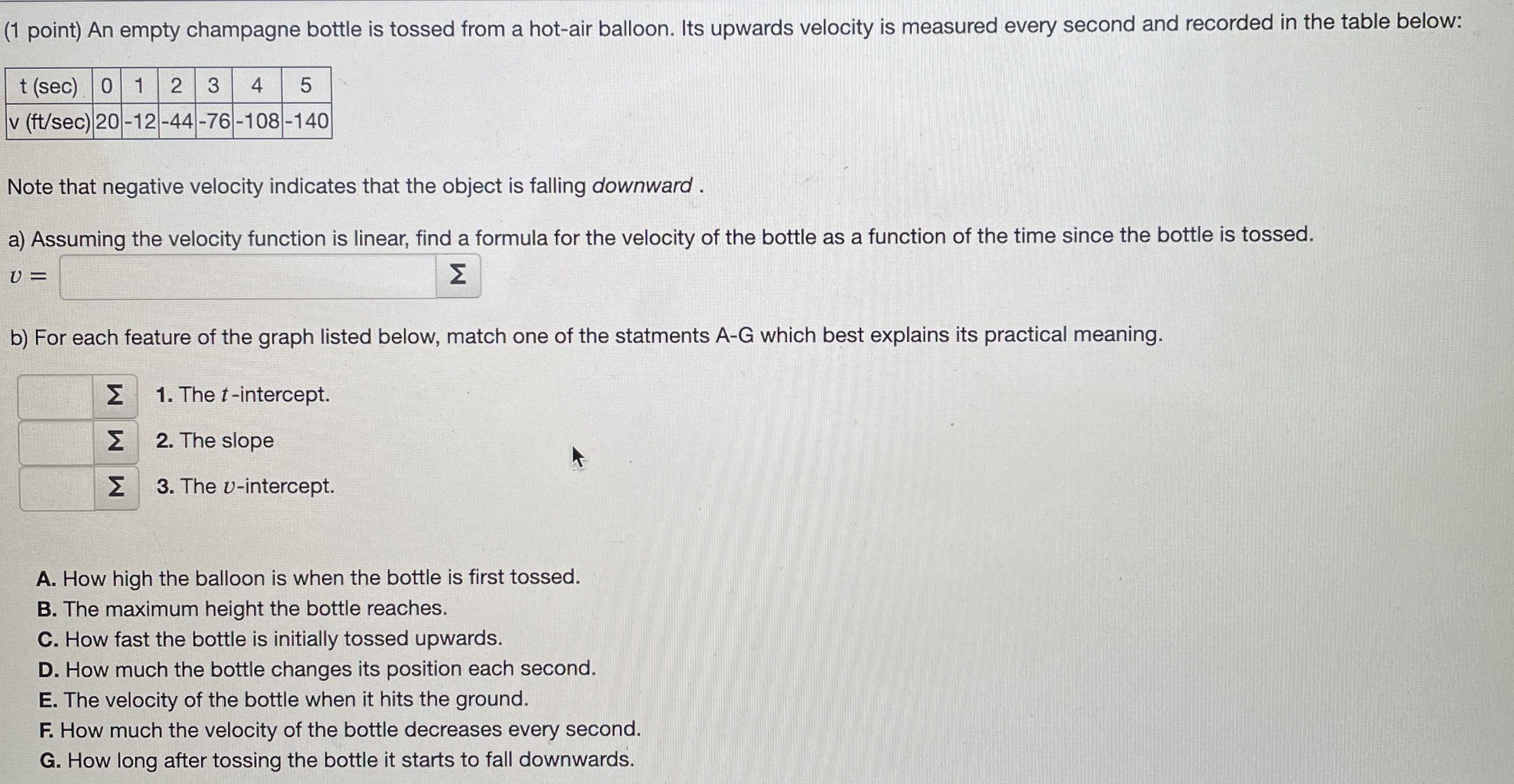### ¿Todavía tienes preguntas de matemáticas?

Pregunte a nuestros tutores expertos
Algebra
Pregunta(1 point) An empty champagne bottle is tossed from a hot-air balloon. Its upwards velocity is measured every second and recorded in the table below:

Note that negative velocity indicates that the object is falling downward.

a) Assuming the velocity function is linear, find a formula for the velocity of the bottle as a function of the time since the bottle is tossed.

$$v =$$ $$\square$$

b) For each feature of the graph listed below, match one of the statments A-G which best explains its practical meaning.

$$\square$$ 1. The $$t$$ -intercept.

$$\square$$ 2. The slope

$$\square$$ 3. The $$v$$ -intercept.

A. How high the balloon is when the bottle is first tossed.

B. The maximum height the bottle reaches.

C. How fast the bottle is initially tossed upwards.

D. How much the bottle changes its position each second.

E. The velocity of the bottle when it hits the ground.

F. How much the velocity of the bottle decreases every second.

G. How long after tossing the bottle it starts to fall downwards.

$$v= 20- 32t$$